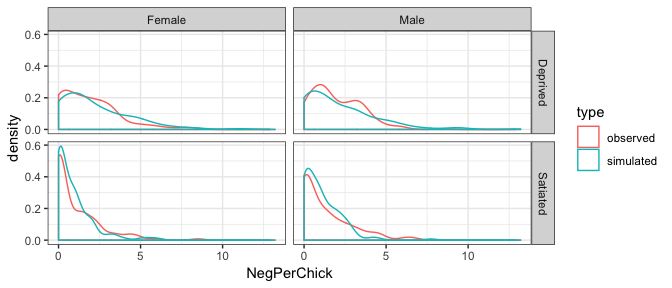# Simulate from a fitted glmmTMB model

#### 2019-01-11

glmmTMB has the capability to simulate from a fitted model. These simulations resample random effects from their estimated distribution. In future versions of glmmTMB, it may be possible to condition on estimated random effects.

library(glmmTMB)
library(ggplot2); theme_set(theme_bw())

Fit a typical model:

data(Owls)
owls_nb1 <- glmmTMB(SiblingNegotiation ~ FoodTreatment*SexParent +
(1|Nest)+offset(log(BroodSize)),
family = nbinom1,
ziformula = ~1, data=Owls)

Then we can simulate from the fitted model with the simulate.glmmTMB function. It produces a list of simulated observation vectors, each of which is the same size as the original vector of observations. The default is to only simulate one vector (nsim=1) but we still return a list for consistency.

simo=simulate(owls_nb1, seed=1)
Simdat=Owls
Simdat$SiblingNegotiation=simo[] Simdat=transform(Simdat, NegPerChick = SiblingNegotiation/BroodSize, type="simulated") Owls$type = "observed"
Dat=rbind(Owls, Simdat) 

Then we can plot the simulated data against the observed data to check if they are similar.

ggplot(Dat,  aes(NegPerChick, colour=type))+geom_density()+facet_grid(FoodTreatment~SexParent)